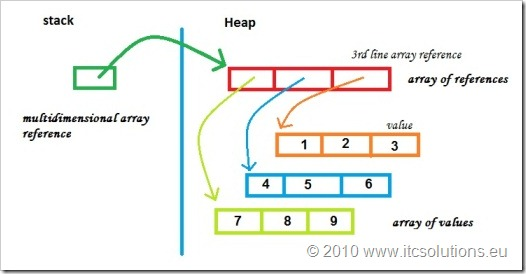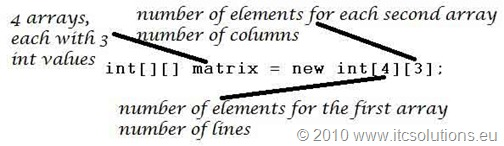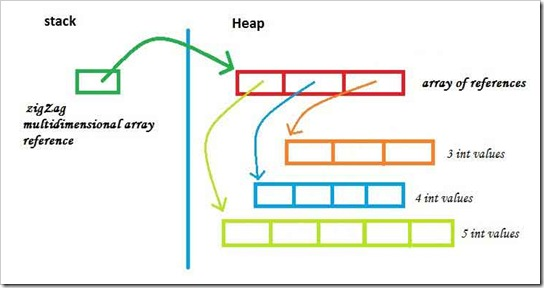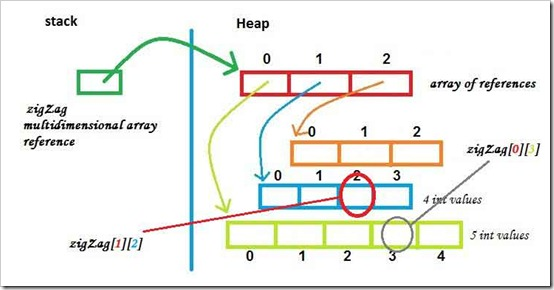# Tutorial Java 6 – #4.3 Matrixes and Multidimensional Arrays

In this post we will see what are and how to define multidimensional arrays. The most common multidimensional array is the matrix – a two dimension array. Despite the fact in our mind a two-dimension array is like a table, a matrix is in Java an array of arrays, like in this image (a two-dimension array with 3 columns and 3 lines):Multidimensional Array in Memory

#### How to define a multidimensional array

The syntax for defining a two-dimensional array in Java:

base_type[][] matrix_name;

base_type matrix_name[][]; // style similar to C/C++

Important! By definition, matrix_name is an uninitialized variable of type reference (pointer) with a default value equal to null. null is a Java keyword which indicates a nonexistent address with value equal to 0.

```        //define a two-dimension matrix
// Java recomended sintax
int[][] matrixExample1;
// C and C++ like sintax
long matrixExample2[][];```

You could also define a three-dimensional array, like a cube:

```        //define a three-dimension matrix
int[][][] cube;```

#### How to initialize multidimensional arrays in Java

Because they are objects, the multidimensional array values are stored in HEAP (an area of RAM memory with dynamic allocation in which processes can reserve space at run-time).

There are 2 ways to initialize a multidimensional array in Java:

• initialize in one operation the space for the entire multidimensional array;
• initialize the size of at least the first dimension (the most significant dimension of the array); after that, in separate operations, there are initialized the less significant dimensions;

On the first approach, the entire multidimensional array is created and initialized (with default values) with a single new instruction:

```        //define a matrix with 3 lines and 3 columns
int[][] matrix3x3;
//create
matrix3x3 = new int;```

When defining a matrix like that, the dimensions represent:On the second approach, an multidimensional array (two dimensions) is initialized in 4 steps:

1. Define the array of arrays (the lines of the matrix);
2. Reserve space for it; initialize the size of the first dimension
3. Reserve space (construct) for each array that will store the values; because each array is processed separately, it is possible to set different sizes and create a zig-zag or jagged matrix;
4. Initialize items value (optional, because during the memory allocation, the elements get default values associated with the base type of the array – for primitive types check How to define primitive data types variables and for references the default value is null)
```        //define a matrix with 3 lines and 3 columns
int[][] matrix3x3;
//create the array of arrays with 3 references
matrix3x3 = new int[];
//create each of the 3 secondary arrays
//the first line or array
matrix3x3 = new int;
//the second line
matrix3x3 = new int;
//the third line
matrix3x3 = new int;```

#### How to define a zig-zag or jagged matrix in Java

For example, we define a multidimensional array that has 3 arrays an each array has 3, 4 and 5 integer values:

```        //define a zig-zag matrix with 3 lines
int[][] zigZag;
//create the array of arrays with 3 references
zigZag = new int[];
//create each of the 3 secondary arrays
//the first line or array
zigZag = new int;
//the second line
zigZag = new int;
//the third line
zigZag = new int;```

and this zig-zag matrix will look in memory like this:Zig-Zag Multidimensional Array

#### How to access and process multidimensional arrays in Java

Like in the case of simple arrays, access to multidimensional array elements is done using operator [], but you must indicate the index for each dimension. ( WARNING! the index used to access the elements has values in the set [0, length-1] for each dimension).

The number of items in an array is determined by accessing its attribute length (in Java, arrays are objects of type array).

```        //initialize the first line elements with value 3
for(int j = 0; j < zigZag.length; j++)
zigZag[j] = 3;
//initialize the second element of the second line with 10
zigZag = 10;

//determine the sum of its elements
int sum = 0;
for(int i = 0; i < zigZag.length; i++)
for(int j = 0; j < zigZag[i].length; j++)
sum += zigZag[i][j];

System.out.println(sum);```Access into a Multidimensional Array

WARNING! the index used to access the elements takes values in the set [0, length-1]. If the index is not managed properly and gets values that exceeds the array length, then you get run-time exceptions like ArrayIndexOutOfBoundsException.

```        //run-time exception ArrayIndexOutOfBoundsException
zigZag = 100;```

Other topics that are part of this Java 6 tutorial are accessible through Tutorial Java 6 – Contents.